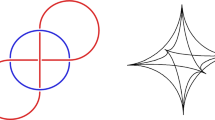# Representations of groups with CAT(0) fixed point property

## Abstract

We show that certain representations over fields with positive characteristic of groups having CAT$$(0)$$ fixed point property $$\mathrm{F}\mathcal {B}_{\widetilde{A}_n}$$ have finite image. In particular, we obtain rigidity results for representations of the following groups: the special linear group over $${\mathbb {Z}}$$, $${\mathrm{SL}}_k({\mathbb {Z}})$$, the special automorphism group of a free group, $$\mathrm{SAut}(F_k)$$, the mapping class group of a closed orientable surface, $$\mathrm{Mod}(\Sigma _g)$$, and many other groups. In the case of characteristic zero, we show that low dimensional complex representations of groups having CAT$$(0)$$ fixed point property $$\mathrm{F}\mathcal {B}_{\widetilde{A}_n}$$ have finite image if they always have compact closure.

This is a preview of subscription content, log in via an institution to check access.

Price excludes VAT (USA)
Tax calculation will be finalised during checkout.

### Lifting images of standard representations of symmetric groups

Jeffrey Yelton### On SL(3, $${\varvec{\mathbb {C}}}$$ )-representations of the Whitehead link group

Antonin Guilloux & Pierre Will

A. N. Panov

## References

1. Abramenko, P., Brown, K.S.: Buildings: Theory and Applications. Graduate Texts in Mathematics, vol. 248. Springer, New York (2008)

2. Alperin, R.: Two-dimensional representations of groups with property FA. Proc. Am. Math. Soc. 108, 283–284 (1990)

3. Ash, R.B.: A Course in Algebraic Number Theory. Dover Books on Mathematics, Mineola (2010)

4. Barnhill, A.: The $$FA_n$$ conjecture for Coxeter groups. Algebr. Geom. Topol. 6, 2117–2150 (2006)

5. Bridson, M.R., Haefliger, A.: Metric Spaces of Non-positive Curvature. Grundlehren der Mathematischen Wissenschaften, vol. 319. Springer, Berlin (1999)

6. Bridson, M.R.: On the dimension of CAT(0) spaces where mapping class groups act. J. Reine Angew. Math. 673, 55–68 (2012)

7. Button, J.O.: Mapping class groups are not linear in positive characteristic. arXiv:1610.08464

8. Farb, B.: Group actions and Helly’s theorem. Adv. Math. 222, 1574–1588 (2009)

9. Grothendieck, A.: Éléments de géométrie algébrique. II. Étude globale élémentaire de quelques classes de morphismes. (French) Inst. Hautes Études Sci. Publ. Math. No. 8 (1961)

10. Humphreys, J.E.: Reflection Groups and Coxeter Groups. Cambridge Studies in Advanced Mathematics, vol. 29. Cambridge University Press, Cambridge (1990)

11. Lam, T.Y.: A First Course in Noncommutative Rings. Graduate Texts in Mathematics, vol. 131, 2nd edn. Springer, New York (2001)

12. Ronan, M.: Lectures on Buildings. University of Chicago Press, Chicago (2009)

13. Schur, I.: Über Gruppen periodischer linearer, Substitutionen, pp. 619–627. Sitzungsberichte der königlich Preussischen Akademie der Wissenschaften, Berlin (1911)

14. Serre, J.-P.: Trees. Springer Monographs in Mathematics. Springer, Berlin (2003)

15. Varghese, O.: Actions of SAut($$F_{n}$$). Arch. Math. 110, 319–225 (2018)

## Acknowledgements

The author would like to thank the referee for many helpful comments.

## Author information

Authors

### Corresponding author

Correspondence to Olga Varghese.